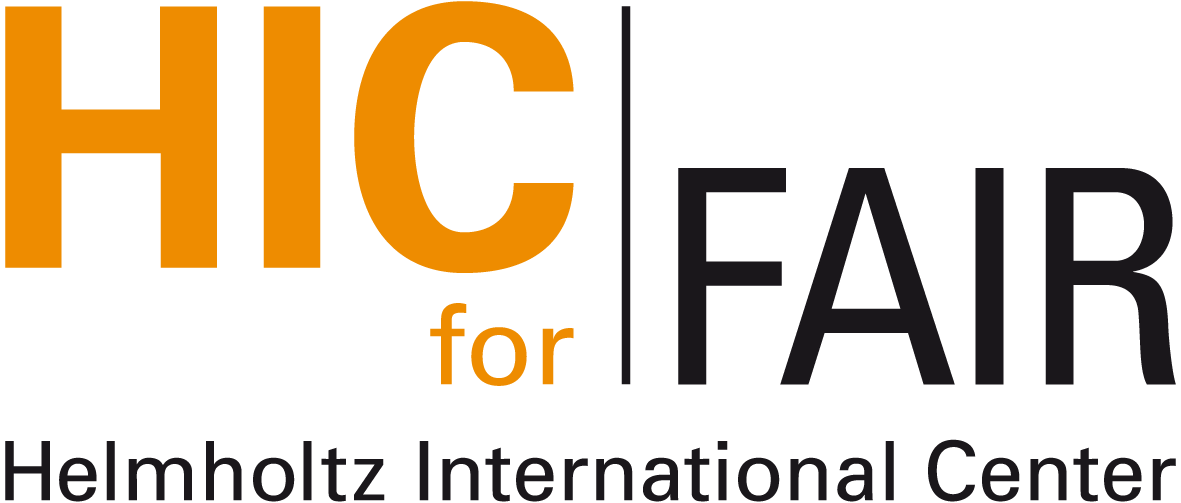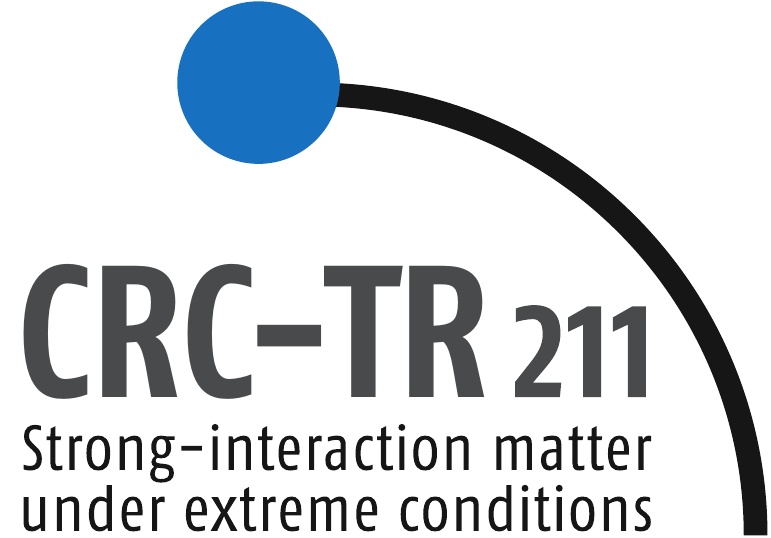#Nuclear Physics Colloquium

Venue: Physics Building, Max-von-Laue-Str. 1, PHYS 02.116
Time: Thursday, December 05, 4:30pm (s.t.)
Contact: hees@itp.uni-frankfurt.de

# Particle momentum spectra and correlations from quasi equilibrium state of expanding quantum fields

## Sergii Akkelin (Bogolyubov Institute for Theoretical Physics, Kiev)

In this talk I discuss particle momentum spectra and correlations from a quasi equilibrium state of the longitudinally boost-invariant expanding quantum field which is locally restricted to the light cone with beginning at the $t = z = 0$ plane of the Minkowski spacetime manifold. I show that the lowest energy eigenstate of the corresponding quasi equilibrium statistical operator does not coincide with the Minkowski vacuum.  Then, I demonstrate results of calculations of one- and two-particle momentum spectra in an exactly solvable model of non-interacting scalar field. The physical picture which follows from these results is that particles are produced by the emitter with two different scales approximately attributed to the expanding ideal gas in the local equilibrium state and to the highly entangled (squeezed) state (condensate) of correlated pairs of particles.  Signals of particle production from such a condensate can be detected if the rate of matter expansion is high, and if the effect of rescatterings for produced particles is small. I suggest that recent experimental observations of the suppression of two-pion Bose-Einstein momentum correlations in the high-multiplicity pp collisions at the LHC can be associated with the ground-state condensate contribution to particle momentum spectra and correlations.

Nuclear Physics Colloquium Homepage